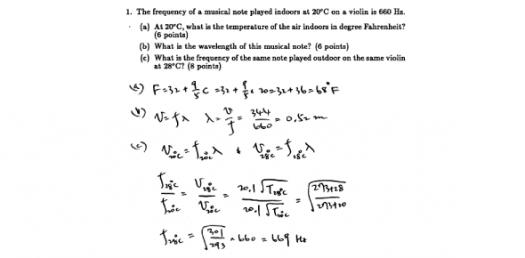# Quiz: Are You Ready To Take The Physics Midterm Exam Test?

19 Questions | Attempts: 1008Settings.

• 1.
Which of Newton's laws best explains why motorists should buckle-up?
• A.

First law

• B.

Second law

• C.

Third law

• D.

Law of Universal Gravitation

• 2.
When you sit on a chair, the resultant force on you is:
• A.

Zero

• B.

Up

• C.

Down

• D.

• 3.
In the absence of an external force, a moving object will:
• A.

Stop immediatly

• B.

Slow down and eventually come to a stop

• C.

Go faster and faster

• D.

Move with constatn velocity

• 4.
You are standing in a moving bus, facing forward, and you suddenly fall forward. You can imply from this that the bus is:
• A.

Velocity increased

• B.

Velocity decreased

• C.

Speed remained the same, but it's turning right.

• D.

speed remained the same, but it's turning left.

• 5.
The acceleration of an object is inversely proportional to:
• A.

The net force acting on it

• B.

Its velocity

• C.

Its mass

• D.

Its position

• 6.
A net force F accelerates a mass m with an acceleration a. If the same net force is applied and the mass is doubled, then the acceleration will be:
• A.

4a

• B.

2a

• C.

A/2

• D.

A/4

• 7.
When the rocket engines on the starship NO-PAIN-NO-GAIN are suddenly turned off, while traveling in empty space, the starship will:
• A.

Stop immediatly

• B.

Slowly slow down, and then stop

• C.

Move with constant speed

• D.

Go faster and faster

• 8.
A rocket moves through empty space in a straight line with constant speed. It is far from the gravitational effect of any star or planet. Under these conditions, the force that must be applied to the rocket in order to sustain its motion is:
• A.

Equal to its weight

• B.

Zero

• C.

Equal to its mass

• D.

Dependent on how fast is going

• 9.
A net force F acts on a mass m and produces an acceleration a. What acceleration results if the force is doubled and it acts on four times the mass?
• A.

8a

• B.

4a

• C.

2a

• D.

A/2

• 10.
If you blow up a balloon, and then release it, the balloon will fly away. This is an illustration of:
• A.

Newton's first law

• B.

Newton's second law

• C.

Newton's third law

• D.

Galileo's law of inertia

• 11.
Two cars collide head-on. At every moment during the collision, the magnitude of the force the first car exerts on the second is exactly equal to the magnitude of the force the second car exerts on the first. This is an example of:
• A.

Newton's first law

• B.

Newton's second law

• C.

Newton's third law

• D.

Law of Universal Gravitation

• 12.
Action-reaction forces:
• A.

Sometimes act on the same object.

• B.

Always act on different objects.

• C.

Always act on the same object.

• D.

May be at right angles

• 13.
Action-reaction forces are:
• A.

Equal magnitude and point in the same direction

• B.

Unequal magnitude, point in the same direction

• C.

equal magnitude but point in opposite directions

• D.

Unequal magnitude, point in opposite directions

• 14.
A 20-ton truck collides with a 1500-lb car and causes a lot of damage to the car. Since a lot of damage is done on the car:
• A.

Force on the truck is smaller than the force on the car

• B.

The truck did not slow down during the collision

• C.

Force on truck is greater than force on the car

• D.

force on the truck is equal to the force on the car

• 15.
An object of mass m sits on a flat table. The Earth pulls on this object with force mg, which we will call the action force. What is the reaction force?
• A.

The object pushing down on the table with force mg.

• B.

The table pushing up on the object with force mg.

• C.

The object pulling upward on the Earth with force mg.

• D.

The table pushing down on the floor with force mg.

• 16.
A child's toy is suspended from the ceiling by means of a string. The Earth pulls downward on the toy with its weight force of 8.0 N. If this is the "action force," what is the "reaction force"?
• A.

The string pulling down on the ceiling with an 8N force.

• B.

The toy pulling up on the Earth with an 8N force.

• C.

The string pulling up on the toy with an 8N force.

• D.

The ceiling pulling up on the string with an 8N force.

• 17.
Mass and weight:
• A.

Are both measured in kilograms.

• B.

Are exactly equal.

• C.

Are two different quantities.

• D.

Both measure the same thing.

• 18.
A constant net force acts on an object. Describe the motion of the object.
• A.

Constant speed

• B.

Constant acceleration

• C.

Constant velocity

• D.

Increasing acceleration

• 19.
Type question here. Example: Practice makes you [Blank]

## Related TopicsBack to top
×

Wait!
Here's an interesting quiz for you.